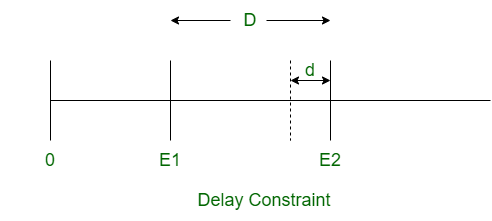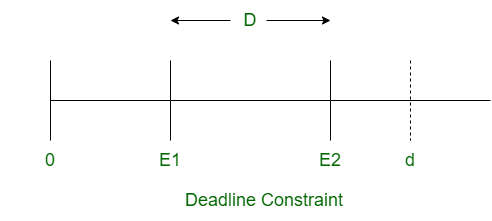# Difference between Delay and Deadline Constraint in Real-time System

• Last Updated : 02 Jun, 2020

Prerequisite – Timing Constraints in Real-time System
1. Delay Constraint :
Delay constraint is the minimum time interval between occurrence of two consecutive events in the real-time system. If an event occurs before the delay constraint, then it is called a delay violation. The time interval between occurrence of two events should be greater than or equal to delay constraint.
If D is the actual time interval between occurrence of two events and d is the delay constraint, then D >= d.Deadline constraint is the maximum time interval between occurrence of two consecutive events in the real-time system. If an event occurs after the deadline constraint, then the result of event is considered incorrect. The time interval between occurrence of two events should be less than or equal to deadline constraint.
If D is the actual time interval between occurrence of two events and d is the deadline constraint, then D <= d.Difference between Delay and Deadline Constraint: